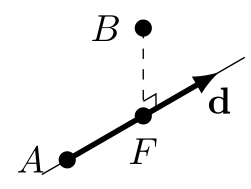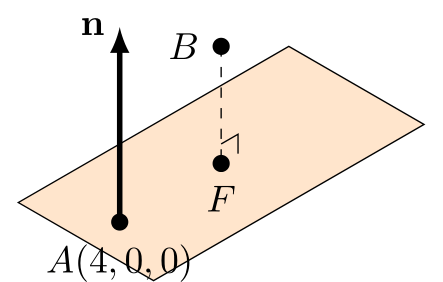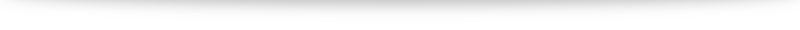# Alternative method to find food of perpendicular (without using vector projections)

In my video (link to be updated) I have worked out examples of how to use projection vectors to find the foot of perpendicular (for both lines and planes). The benefits of this method

1. it is faster,
2. it uses a similar concept for both lines and planes with slight modifications.

The downsides, however, is that it involves a rather unwieldy projection vector formula $(\mathbf{a}\cdot\mathbf{\hat{b}}) \mathbf{\hat{b}}$ and that minor details make a huge difference to whether we get the correct answer (e.g. where are our vectors pointing, $\overrightarrow{AB}$ or $\overrightarrow{BA}$? Is our final projection vector $\overrightarrow{AF}$ or $\overrightarrow{FB}$?).
In this post I will offer an alternative method to find foot of perpendicular using earlier concepts that some students find easier to understand.

### Finding foot of perpendicular to a lineWe will use the example of finding the foot of perpendicular, $F$ from $B(4,6,-1)$ to the line $$l: \mathbf{r} = \begin{pmatrix} -1 \\ 5 \\ -3 \end{pmatrix} + \lambda \begin{pmatrix} 1 \\ -1 \\ 1 \end{pmatrix}, \lambda \in \mathbb{R}.$$

There are two key ideas behind this approach:

1. $F$ lies on the line: hence we can use the equation of the line
2. $BF$ is perpendicular to the line: hence $\overrightarrow{BF}\cdot \mathbf{d} = 0$.

Since $F$ lies on the line, we can use the equation of the line and write $$\overrightarrow{OF} = \begin{pmatrix} -1+\lambda \\ 5-\lambda \\ -3+\lambda \end{pmatrix}$$ We next find $\overrightarrow{BF} = \overrightarrow{OF} - \overrightarrow{OB} = \begin{pmatrix} -5+\lambda \\ 1-\lambda \\ 2+\lambda \end{pmatrix}$.
Since $BF$ is perpendicular to the line, $\overrightarrow{BF}$ is perpendicular to the direction vector of the line. \begin{gather} \overrightarrow{BF} \cdot \mathbf{d} = 0 \\ \begin{pmatrix} -5+\lambda \\ -1-\lambda \\ -2+\lambda \end{pmatrix} \cdot \begin{pmatrix} 1 \\ -1 \\ 1 \end{pmatrix} = 0 \\ -6+3\lambda = 0 \\ \lambda = 2 \end{gather} Substituting that back into the equation for $\overrightarrow{OF}$ gives us $\overrightarrow{OF} = \begin{pmatrix} 1 \\ 3 \\ -1 \end{pmatrix}$.

### Finding foot of perpendicular to a planeWe will use the example of finding the foot of perpendicular, $F$ from $B(4,6,-1)$ to the plane $$p: \mathbf{r} \cdot \begin{pmatrix} 1 \\ 1 \\ 0 \end{pmatrix} = 4.$$

There are two key ideas behind this approach:

1. We will form the equation of the line $BF$, noting that it is parallel to the normal vector of the plane.
2. The intersection point between the line $BF$ and the plane is the foot of perpendicular $F$.

To form an equation for the line $BF$, we note that $B$ lies on the line and the normal vector of the plane is the direction vector of the line. Thus $$l_{BF}: \mathbf{r} = \begin{pmatrix} 4 \\ 6 \\ -1 \end{pmatrix} + \lambda \begin{pmatrix} 1 \\ 1 \\ 0 \end{pmatrix}, \lambda \in \mathbb{R}.$$ Take note to not confuse the line $BF$ and the vector $\overrightarrow{BF}$.

$F$ is the point of intersection between $l_{BF}$ and the plane $p$. We thus substitute the equation of the line into the plane: \begin{gather} \begin{pmatrix} 4+\lambda \\ 6+\lambda \\ -1 \end{pmatrix} \cdot \begin{pmatrix} 1 \\ 1 \\ 0 \end{pmatrix} = 4 \\ 10+2\lambda = 4 \\ \lambda = -3 \end{gather} Substituting that back into the equation of the line gives us $\overrightarrow{OF} = \begin{pmatrix} 1 \\ 3 \\ -1 \end{pmatrix}$.